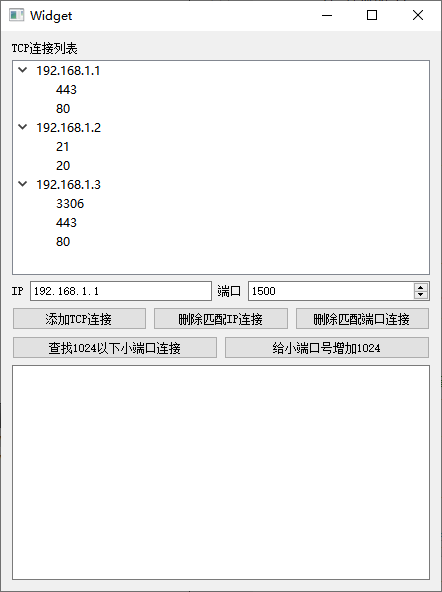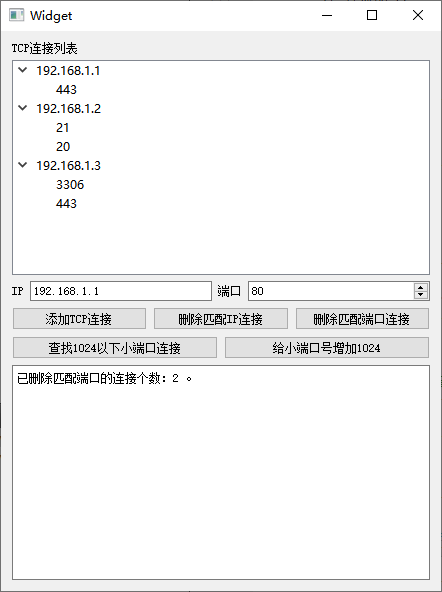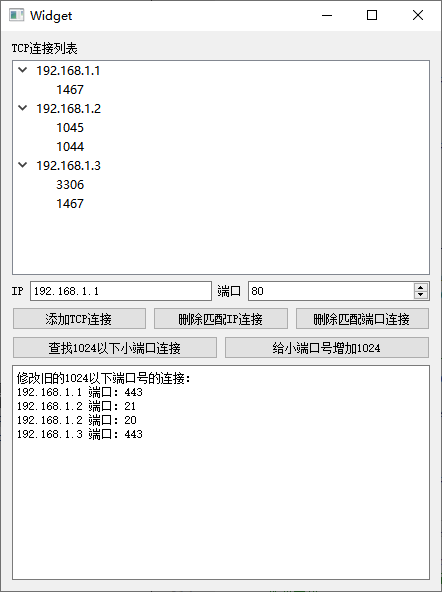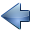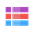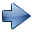9.5 数据容器的迭代器

9.5.1 STL 风格迭代器

 容器 只读迭代器 读写迭代器 QList, QQueue QList::const_iterator QList::iterator QLinkedList QLinkedList::const_iterator QLinkedList::iterator QVector, QStack QVector::const_iterator QVector::iterator QMap, QMultiMap QMap::const_iterator QMap::iterator QHash, QMultiHash QHash::const_iterator QHash::iterator QSet QSet::const_iterator QSet::iterator

QList<QString> list;
list << "A" << "B" << "C" << "D";

QList<QString>::iterator i;
for (i = list.begin(); i != list.end(); ++i)
*i = (*i).toLower();

STL 迭代器遍历过程如下图所示：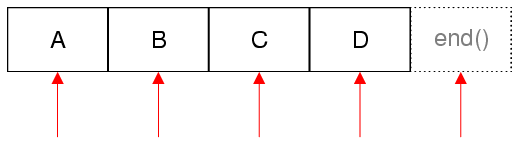QMap<int, int> map;
map.insert(1,1);
map.insert(2,4);
map.insert(3,9);
//迭代访问
QMap<int, int>::const_iterator it;
for(it=map.constBegin(); it!=map.constEnd(); ++it)
{
qDebug()<< it.key() << it.value();
qDebug()<< *it;
}
it.key() 返回元素的关键字内容，it.value() 返回映射值内容，映射和哈希容器也有 *it 的用法。 *it 等同于 it.value() 映射值。上述代码输出为：
1 1
1
2 4
4
3 9
9
STL 风格迭代器常见运算符使用如下表所示：
 表达式 行为 *i 访问元素的数值(映射类元素的 value) ++i 移动到下一个元素，注意该运算符不检查越界，越界就是野指针 i += n 移动到后面第 n 个元素，注意该运算符不检查越界，越界就是野指针 --i 移动到前一个元素，注意该运算符不检查越界，越界就是野指针 i += n 移动到前面第 n 个元素，注意该运算符不检查越界，越界就是野指针 i - j 对于顺序容器，计算两个迭代器 i 和 j 位置中间包括元素的个数； 如果 i 是迭代器，j 是 int，返回迭代器 i 往前第 j 个元素迭代器。 对于关联容器，j 不能是迭代器，j 只能是整数int，返回迭代器 i 往前第 j 个元素迭代器。 i.key() 和 i.value() 映射类元素的关键字 key 和映射值 value

--i 、++i 与 i--、i++ 都类似指针的用法，前一对在表达式计算之前增减数值，后一对在表达式计算之后增减数值，通常推荐使用 --i 、++i ，这对操作执行效率稍高一些。

QList<QString> list;
list << "A" << "B" << "C" << "D";

QList<QString>::iterator i = list.end();
while ( i != list.begin() )
{
--i;
*i = (*i).toLower();
}

`    QVector<int> a, b;`
`    a.resize(100); //向量初始化为100个0`
`    // i 指向向量第一个元素`
`    QVector<int>::iterator i = a.begin();`
`    //隐式共享，b 和 a 指向同一块存储空间`
`    b = a;`
`    //隐式共享在一个对象发生变化后，为变化的对象分配新空间，并赋值`
`    // a 元素修改后，i 迭代器与 a 无关了！！！`
`    // i 其实指向 b 首元素，因为 b 没有修改，使用旧的内存空间`
`    a = 5;`
`    //这时候 *i 是 b 开头的数值 0`
`    b.clear(); //清空 b，那么迭代器 i 就属于野指针！！！`

`    // 迭代器的错误示范`
`    int j = *i; //野指针使用，未知结果`

// OK
const QList<int> sizes = splitter->sizes();
QList<int>::const_iterator i;
for (i = sizes.begin(); i != sizes.end(); ++i)
...

QHash<QString, int>::iterator i = hash.begin();
while (i != hash.end())
{
if (i.key().startsWith("_"))
i = hash.erase(i);
else
++i;
}

QHash<QString, int>::iterator i = hash.begin();
while (i != hash.end())
{
QHash<QString, int>::iterator prev = i;
++i;
if (prev.key().startsWith("_"))
hash.erase(prev);
}

①项目名称 salary，创建路径 D:\QtProjects\ch09，点击下一步；
②套件选择里面选择全部套件，点击下一步；
③基类选择 QWidget，点击下一步；
④项目管理不修改，点击完成。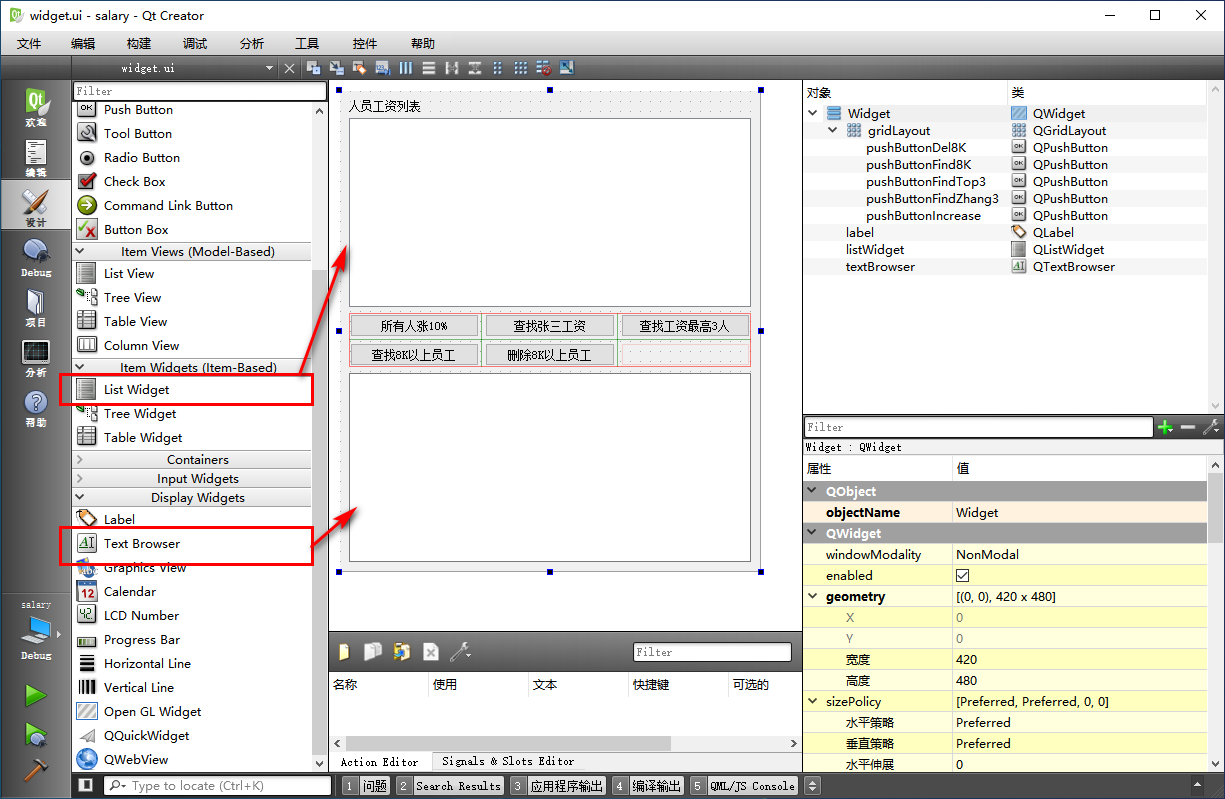#ifndef WIDGET_H
`#define WIDGET_H`

`#include <QWidget>`
`#include <QMultiHash>`
`#include <QMultiMap> //可以用于键值对的同步排序`

`namespace Ui {`
`class Widget;`
`}`

`class Widget : public QWidget`
`{`
`    Q_OBJECT`

`public:`
`    explicit Widget(QWidget *parent = 0);`
`    ~Widget();`

`    //初始化填充`
`    void InitSalary();`
`    //工资列表变动时，显示到列表控件`
`    void UpdateSalaryShow();`

`private slots:`
`    void on_pushButtonIncrease_clicked();`

`    void on_pushButtonFindZhang3_clicked();`

`    void on_pushButtonFindTop3_clicked();`

`    void on_pushButtonFind8K_clicked();`

`    void on_pushButtonDel8K_clicked();`

`private:`
`    Ui::Widget *ui;`
`    //使用哈希映射保存工资表`
`    QMultiHash<QString, double> m_salary;`
`};`

`#endif // WIDGET_H`

UpdateSalaryShow() 函数用于在成员变量 m_salary 变动时，更新列表控件显示。其他代码都是自动生成的，包括 5 个槽函数。

#include "widget.h"
`#include "ui_widget.h"`
`#include <QMessageBox>`
`#include <QDebug>`

`Widget::Widget(QWidget *parent) :`
`    QWidget(parent),`
`    ui(new Ui::Widget)`
`{`
`    ui->setupUi(this);`
`    //初始化工资表`
`    InitSalary();`
`    //更新显示`
`    UpdateSalaryShow();`
`}`

`Widget::~Widget()`
`{`
`    delete ui;`
`}`

`//初始化填充`
`void Widget::InitSalary()`
`{`
`    m_salary.clear();//清空`
`    //添加工资内容`
`    m_salary.insert(tr("张三"), 5000.0);`
`    m_salary.insert(tr("张三"), 8000.0); //有重名的`
`    m_salary.insert(tr("李四"), 6000.0);`
`    m_salary.insert(tr("王五"), 7000.0);`
`    m_salary.insert(tr("孙六"), 8000.0);`
`    m_salary.insert(tr("赵七"), 6600.0);`
`    m_salary.insert(tr("钱八"), 8800.0);`
`}`

`//工资列表变动时，显示到列表控件`
`void Widget::UpdateSalaryShow()`
`{`
`    //清空旧的内容`
`    ui->listWidget->clear();`
`    //只读迭代器遍历`
`    QMultiHash<QString, double>::const_iterator it;`
`    for( it=m_salary.constBegin(); it!=m_salary.constEnd(); ++it )`
`    {`
`        QString strLine = it.key() + tr("\t%1").arg( it.value() );`
`        ui->listWidget->addItem( strLine );`
`    }`
`    //完成`
`}`

//让所有人涨工资 10%
`void Widget::on_pushButtonIncrease_clicked()`
`{`
`    //读写迭代器遍历`
`    QMultiHash<QString, double>::iterator it;`
`    for( it=m_salary.begin(); it!=m_salary.end(); ++it)`
`    {`
`        //读写迭代器 it.value() 返回可以读写的 value 引用，可以作为左值写入`
`        it.value() *= 1.1 ;`
`        //注意 it.key() 永远是只读引用，不能修改key，映射的 key 只能删除或添加，不能直接修改`
`    }`
`    //更新显示`
`    UpdateSalaryShow();`
`    ui->textBrowser->setText( tr("所有人涨工资 10% 完毕。") );`
`}`

it.value() *= 1.1;
这句代码就是能够直接修改元素的 value 值，变为原来的 1.1 倍，就是涨工资 10% ，处理每个元素后，迭代器指向末尾的 m_salary.end()，结束循环。

//查找所有叫张三的人工资
`void Widget::on_pushButtonFindZhang3_clicked()`
`{`
`    //张三有重名，找到第一个张三`
`    QMultiHash<QString, double>::const_iterator it;`
`    it = m_salary.find( tr("张三") );`
`    //信息字符串`
`    QString strInfo = tr("查找张三结果：\r\n");`
`    //查找多个张三`
`    while( it != m_salary.constEnd() )`
`    {`
`        if( it.key() == tr("张三") ) //连续的多个张三都显示`
`        {`
`            strInfo += it.key() + tr(" 工资： %1\r\n").arg( it.value() );`
`            ++it; //继续找下一个`
`        }`
`        else //人名不等于张三`
`        {`
`            break;  //遍历到 不是张三的位置，不需要再查找`
`        }`
`    }`
`    //显示`
`    ui->textBrowser->setText( strInfo );`
`}`

//查找工资前三的人
`void Widget::on_pushButtonFindTop3_clicked()`
`{`
`    //检查员工人数，如果不超过三个人，就不处理`
`    if( m_salary.count() <= 3 )`
`    {`
`        ui->textBrowser->setText(tr("不超过三个人，不需要查询。"));`
`        return;`
`    }`
`    //遍历哈希对象，然后创建工资到人名的QMultiMap映射对象`
`    //哈希对象迭代器`
`    QMultiHash<QString, double>::const_iterator it;`
`    //工资到人名的反向映射`
`    QMultiMap<double, QString> mapOrder;`
`    //迭代处理`
`    for( it=m_salary.constBegin(); it!=m_salary.constEnd(); ++it)`
`    {`
`        mapOrder.insert( it.value(), it.key() );//反向映射`
`    }`

`    //QMultiMap 和 QMap 插入节点时，会自动按照 key 值排序，从小到大排序`
`    QString strInfo = tr("工资前三的员工：\r\n");`
`    QMultiMap<double, QString>::const_iterator itMap;`
`    itMap = mapOrder.constEnd(); //注意不能访问 end 虚假节点`
`    //查找最后三个即可`
`    for(int i=0; i<3; i++)`
`    {`
`        --itMap; //开头 --，会跳过 end 虚假节点`
`        strInfo += itMap.value() + tr(" 工资：%1\r\n").arg( itMap.key() );`
`    }`
`    //显示`
`    ui->textBrowser->setText( strInfo );`
`}`

mapOrder 的元素添加完成后，排序也就自动完成了，按照工资从小到大排列，我们只需要读取排在最后的三个，就是工资最高的三个人。

//查找 8K 以上的员工
`void Widget::on_pushButtonFind8K_clicked()`
`{`
`    //哈希对象迭代器`
`    QMultiHash<QString, double>::const_iterator it;`
`    //信息字符串`
`    QString strInfo = tr("查找8K以上工资的员工：\r\n");`
`    for( it=m_salary.cbegin(); it!=m_salary.cend(); ++it )`
`    {`
`        if( it.value() >= 8000 ) //判断 8K 以上的`
`        {`
`            strInfo += it.key() + tr(" 工资：%1\r\n").arg( it.value() );`
`        }`
`    }`
`    //显示`
`    ui->textBrowser->setText( strInfo );`
`}`

//删除 8K 以上员工
`void Widget::on_pushButtonDel8K_clicked()`
`{`
`    //读写迭代器`
`    QMultiHash<QString, double>::iterator it;`
`    //信息字符串`
`    QString strInfo = tr("删除8K以上工资的员工：\r\n");`
`    for( it=m_salary.begin(); it!=m_salary.end(); NULL )`
`    {`
`        if( it.value() >= 8000 ) //判断 8K 以上的`
`        {`
`            strInfo += it.key() + tr(" 工资：%1\r\n").arg( it.value() );`
`            //删除迭代器指向的元素，注意旧的 it 删除了不可用`
`            //必须用 erase() 返回值作为后面元素的新迭代器`
`            it = m_salary.erase( it );`
`        }`
`        else //不删除，直接下一个`
`        {`
`            ++it;`
`        }`
`    }`
`    //更新列表控件`
`    UpdateSalaryShow();`
`    //显示信息字符串`
`    ui->textBrowser->setText( strInfo );`
`}`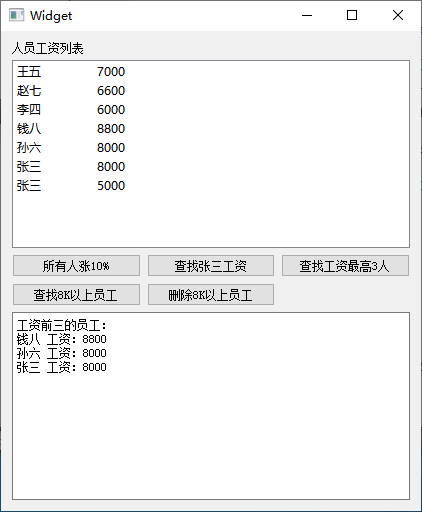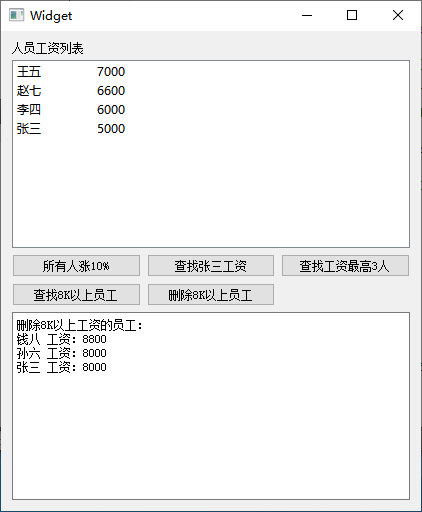9.5.2 Java 风格迭代器

 容器 只读迭代器 读写迭代器 QList, QQueue QListIterator QMutableListIterator QLinkedList QLinkedListIterator QMutableLinkedListIterator QVector, QStack QVectorIterator QMutableVectorIterator QMap, QMultiMap QMapIterator QMutableMapIterator QHash, QMultiHash QHashIterator QMutableHashIterator QSet QSetIterator QMutableSetIterator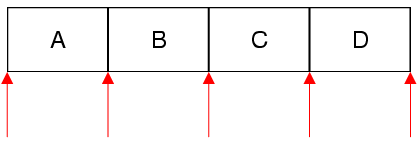Java 风格迭代器的示例代码如下：
QList<QString> list;
list << "A" << "B" << "C" << "D";

QListIterator<QString> i(list);
while (i.hasNext())
qDebug() << i.next();
QListIterator<QString> i(list) 这句代码是根据列表对象定义一个 Java 风格迭代器，这时候迭代器 i 指向首元素前面的狭缝，

QListIterator<QString> i(list);
i.toBack();
while (i.hasPrevious())
qDebug() << i.previous();
i.toBack() 就是移动到末尾元素后面的狭缝，然后使用 i.previous() 函数，访问元素并向前移动；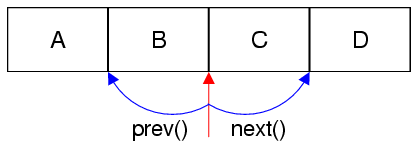函数 行为 void toFront() 移动到首元素前面的狭缝 void toBack() 移动到尾元素后面的狭缝 bool hasNext() const 如果位置不是尾元素后面的狭缝，返回 true Item next() 向后移动一个狭缝位置，并返回狭缝前面的刚滑过的元素 Item peekNext() const 返回狭缝后面的元素，不移动狭缝位置 bool hasPrevious() const 如果不在首元素前面的狭缝位置，返回 true Item previous() 向前移动一个狭缝位置，并返回狭缝后面的刚滑过的元素 Item peekPrevious() const 返回狭缝前面的元素，不移动狭缝。 bool findNext(const T & value) 从迭代器当前狭缝开始向后查找，如果找到匹配 value 的元素，移动到该元素后面的狭缝（正好滑过该元素），返回 true，否则移动到尾元素后面的狭缝，并返回 false bool findPrevious(const T & value) 从迭代器当前狭缝开始向前查找，如果找到匹配 value 的元素，移动到该元素前面的狭缝（正好滑过该元素），返回 true，否则移动到首元素前面的狭缝，并返回 false const Key & key() constconst T &  value() const 映射和哈希映射的迭代器函数，返回关键字和映射值

`    QMap<int, int> map;`
`    map.insert(1,1);`
`    map.insert(2,4);`
`    map.insert(3,9);`
`    //Java 风格迭代器`
`    QMapIterator<int, int> it( map );`
`    while( it.hasNext() )`
`    {`
`        it.next();`
`        qDebug()<<it.key()<<it.value();`
`    }`

`    //要从头开始 findNext()，如果从末尾开始 findNext()那么后面没有任何元素`
`    it.toFront();`
`    //查找`
`    bool bRet = it.findNext( 9 );`
`    if( bRet )`
`        qDebug()<<it.key()<<it.value();`
`    else`
`        qDebug()<<"Can not find.";`

1 1
2 4
3 9
3 9

 函数 行为 void setValue(const T & value) 针对刚滑过的元素，设置 value 值 T & value() 返回刚滑过的元素的 value 的可读写引用，支持 it.value() += 1024 这种修改数值代码。 void remove() 删除刚滑过的元素。

Java 风格迭代器的所有读写操作都是针对刚滑过的元素，而不管迭代器位置是在元素的前面或后面狭缝。例如下面代码：
`    QMap<int, int> map;`
`    map.insert(1,1);`
`    map.insert(2,4);`
`    map.insert(3,9);`
`    //Java 风格读写迭代器`
`    QMutableMapIterator<int, int> it(map);`
`    while(it.hasNext())`
`    {`
`        it.next(); //滑过一个元素`
`        if( it.key() == it.value() )//对刚滑过的元素进行访问和处理`
`            it.remove(); //删除关键字等于映射值的元素`
`        else`
`            qDebug()<<it.key()<<it.value();`
`    }`

2 4
3 9

Java 风格迭代器的内容介绍到这，下面我们学习一个模拟 TCP 连接管理的例子，例子仅使用模拟的 IP 地址和端口号作为数据存在容器里，并不真的进行网络连接。

①项目名称 tcpmanager，创建路径 D:\QtProjects\ch09，点击下一步；
②套件选择里面选择全部套件，点击下一步；
③基类选择 QWidget，点击下一步；
④项目管理不修改，点击完成。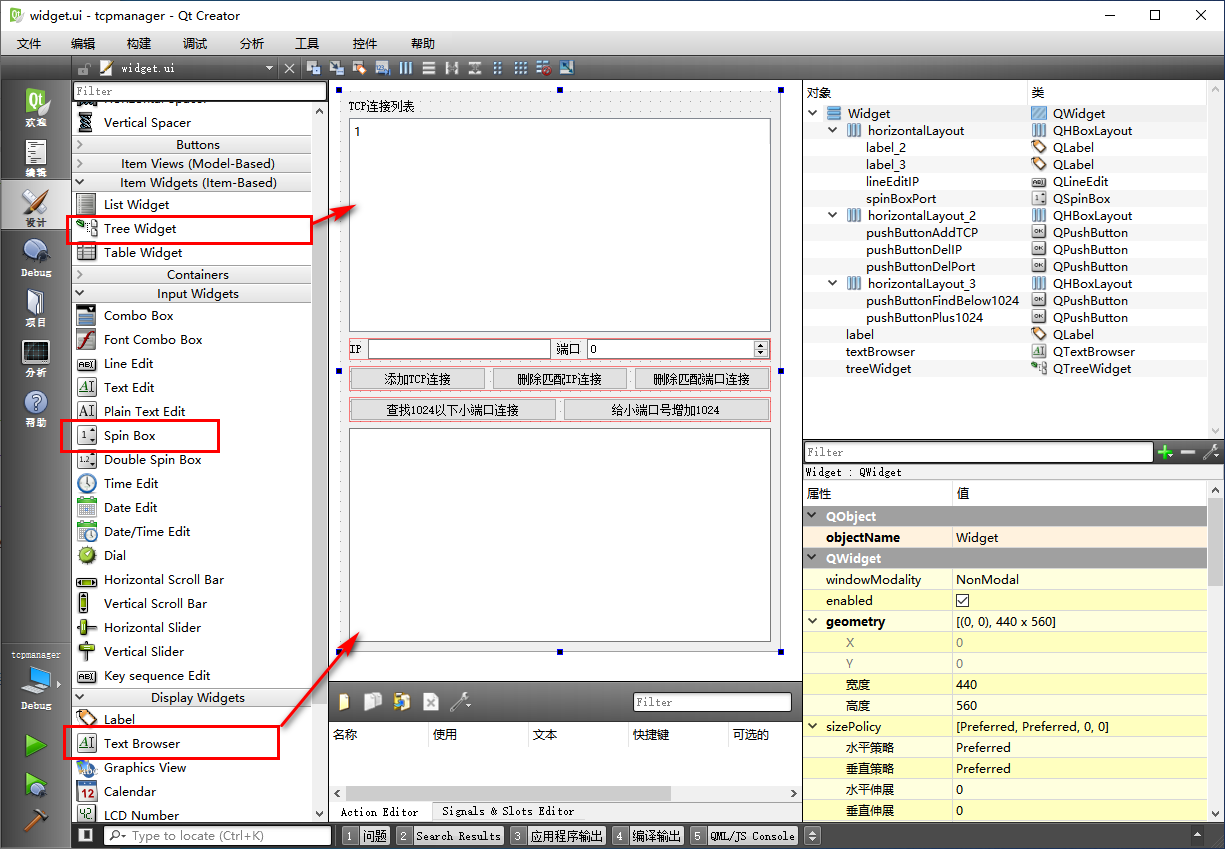#ifndef WIDGET_H
`#define WIDGET_H`

`#include <QWidget>`
`#include <QMultiMap> //保存IP和端口映射`

`namespace Ui {`
`class Widget;`
`}`

`class Widget : public QWidget`
`{`
`    Q_OBJECT`

`public:`
`    explicit Widget(QWidget *parent = 0);`
`    ~Widget();`

`    //初始化填充`
`    void InitTCPLinks();`
`    //更新树形控件的显示`
`    void UpdateTreeShow();`

`private slots:`
`    void on_pushButtonAddTCP_clicked();`

`    void on_pushButtonDelIP_clicked();`

`    void on_pushButtonDelPort_clicked();`

`    void on_pushButtonFindBelow1024_clicked();`

`    void on_pushButtonPlus1024_clicked();`

`private:`
`    Ui::Widget *ui;`
`    //保存IP和端口信息`
`    QMultiMap<QString, int> m_tcplinks;`

`};`

`#endif // WIDGET_H`

#include "widget.h"
`#include "ui_widget.h"`
`#include <QMessageBox>`
`#include <QDebug>`
`#include <QMapIterator>`
`#include <QMutableMapIterator>`
`#include <QTreeWidgetItem>`
`#include <QRegExp>`
`#include <QRegExpValidator>`

`Widget::Widget(QWidget *parent) :`
`    QWidget(parent),`
`    ui(new Ui::Widget)`
`{`
`    ui->setupUi(this);`

`    //设置树形控件只有 1 列`
`    ui->treeWidget->setColumnCount( 1 );`
`    ui->treeWidget->header()->setHidden( true ); //隐藏头部，未使用`
`    //设置 IP编辑框`
`    //定义 IPv4 正则表达式，注意 "\\" 就是一个反斜杠字符`
`    QRegExp re("^(?:(?:25[0-5]|2[0-4][0-9]|?[0-9][0-9]?)\\.){3}"`
`               "(?:25[0-5]|2[0-4][0-9]|?[0-9][0-9]?)\$");`
`    //新建正则表达式验证器`
`    QRegExpValidator *reVali = new QRegExpValidator(re);`
`    //设置给 lineEditIP`
`    ui->lineEditIP->setValidator(reVali);`
`    ui->lineEditIP->setText( tr("192.168.1.1") ); //默认值`
`    //设置端口范围`
`    ui->spinBoxPort->setRange( 0, 65535 );`
`    ui->spinBoxPort->setValue( 1500 ); //默认值`

`    //初始化填充连接信息`
`    InitTCPLinks();`
`    //更新树形控件的显示`
`    UpdateTreeShow();`
`}`

`Widget::~Widget()`
`{`
`    delete ui;`
`}`

`//初始化填充`
`void Widget::InitTCPLinks()`
`{`
`    m_tcplinks.clear(); //清空旧的`
`    m_tcplinks.insert( tr("192.168.1.1"), 80 );`
`    m_tcplinks.insert( tr("192.168.1.1"), 443 );`
`    m_tcplinks.insert( tr("192.168.1.2"), 20 );`
`    m_tcplinks.insert( tr("192.168.1.2"), 21 );`
`    m_tcplinks.insert( tr("192.168.1.3"), 80 );`
`    m_tcplinks.insert( tr("192.168.1.3"), 443 );`
`    m_tcplinks.insert( tr("192.168.1.3"), 3306 );`
`}`

//更新树形控件的显示
`void Widget::UpdateTreeShow()`
`{`
`    ui->treeWidget->clear(); //清空旧的`
`    //定义迭代器`
`    QMapIterator<QString, int> it( m_tcplinks );`
`    //保存迭代中旧的IP`
`    QString strOldIP;`
`    //保存旧的顶级IP节点`
`    QTreeWidgetItem *pOldTopItem = NULL;`
`    //端口号节点`
`    QTreeWidgetItem *pPortItem = NULL;`
`    //迭代遍历`
`    while( it.hasNext() )`
`    {`
`        it.next();  //滑过一个元素`
`        //获取滑过元素的 IP 和端口`
`        QString strIP = it.key();`
`        int nPort = it.value();`
`        //判断`
`        if( strIP != strOldIP )`
`        {`
`            //新的主机IP，建立顶级IP节点`
`            pOldTopItem = new QTreeWidgetItem();`
`            pOldTopItem->setText(0, strIP);`
`            ui->treeWidget->addTopLevelItem( pOldTopItem );`
`            //添加端口号为子节点`
`            pPortItem = new QTreeWidgetItem();`
`            pPortItem->setText( 0, tr("%1").arg(nPort) );`
`            pOldTopItem->addChild( pPortItem );`
`            //更新旧的IP`
`            strOldIP = strIP;`
`        }`
`        else`
`        {`
`            //现在元素IP 与 上一个元素IP一样`
`            //添加 pOldTopItem 子节点`
`            pPortItem = new QTreeWidgetItem();`
`            pPortItem->setText( 0, tr("%1").arg(nPort) );`
`            pOldTopItem->addChild( pPortItem );`
`        }`
`    }// end while`
`    //遍历结束，树形控件条目添加完成`
`    ui->treeWidget->expandAll(); //全部展开`
`}`

比较当前 strIP 与旧的 strOldIP 是否相等，如果不相等，说明遇到新的主机 IP，那么我们新建树形条目，存到 pOldTopItem，设置文本为 strIP，并设置为树形控件新的顶级条目；然后为这个顶级条目新建一个子条目 pPortItem，子条目内容就是该主机 IP  的端口号；然后将新的主机 IP 地址存到 strOldIP，用于下轮循环比较；
如果当前循环的 strIP 等于 strOldIP，那说明还是同一个主机 IP 的端口，那么直接新建一个条目 pPortItem ，内容是端口号，添加为顶级条目 pOldTopItem 的子条目。

//添加一个连接
`void Widget::on_pushButtonAddTCP_clicked()`
`{`
`    //获取 IP 和端口`
`    QString strIP = ui->lineEditIP->text().trimmed();`
`    if( strIP.isEmpty() )`
`    {`
`        ui->textBrowser->setText( tr("IP为空。") );`
`        return; //IP为空`
`    }`
`    //端口`
`    int nPort = ui->spinBoxPort->value();`
`    //检查是否已存在相同的 IP和端口`
`    if( m_tcplinks.contains( strIP, nPort ) )`
`    {`
`        ui->textBrowser->setText( tr("该IP端口的连接已存在，IP和端口不能同时重复。") );`
`        return;`
`    }`
`    //不重复的连接，添加`
`    m_tcplinks.insert( strIP, nPort );`
`    ui->textBrowser->setText( tr("添加TCP连接完成。") );`
`    //更新树形控件`
`    UpdateTreeShow();`
`}`

//删除匹配IP的连接
`void Widget::on_pushButtonDelIP_clicked()`
`{`
`    //获取 IP`
`    QString strIP = ui->lineEditIP->text().trimmed();`
`    if( strIP.isEmpty() )`
`    {`
`        ui->textBrowser->setText( tr("IP为空。") );`
`        return; //IP为空`
`    }`
`    //删除计数`
`    int nDelCount = 0;`
`    //使用迭代器查找`
`    QMutableMapIterator<QString, int> it( m_tcplinks );`
`    //循环查找`
`    while ( it.hasNext() )`
`    {`
`        it.next(); //滑过一个元素`
`        if( it.key() == strIP ) //检查滑过元素的 key`
`        {`
`            //删除刚找到的滑过元素`
`            it.remove();`
`            nDelCount += 1; //更新删除计数`
`        }`
`    }`
`    //判断`
`    if( nDelCount < 1 )`
`    {`
`        ui->textBrowser->setText( tr("没有匹配的IP。") );`
`    }`
`    else`
`    {`
`        ui->textBrowser->setText( tr("已删除匹配IP的连接个数：%1 。").arg( nDelCount ) );`
`        //更新树形控件`
`        UpdateTreeShow();`
`    }`
`}`

先使用 it.next() 滑过一个元素，这个滑过的元素就是要访问的内容；
我们判断滑过元素的 key() ，如果等于 strIP，那么找到匹配的元素，调用  it.remove() 删除刚滑过的元素，并让删除计数加一，进入下轮循环；
如果 key() 不等于 strIP，不处理，直接进入下轮循环。

//删除匹配端口的连接
`void Widget::on_pushButtonDelPort_clicked()`
`{`
`    //获取端口号`
`    const int nFindPort = ui->spinBoxPort->value();`
`    int nDelCount = 0; //删除计数`
`    //读写迭代器`
`    QMutableMapIterator<QString, int> it( m_tcplinks );`
`    //循环迭代`
`    it.toFront(); //从头开始`
`    //注意 Q*MapIterator 迭代器的 findNext() 和 findPrevious() 比较的是 value ;`
`    // 而 QMap/QMultiMap 容器类的 find() 比较的是 key 或者 key-value 对。`
`    while( it.findNext( nFindPort ) ) //端口号是 value，可以用迭代器的查找函数`
`    {`
`        it.remove();`
`        nDelCount += 1;`
`    }`
`    //遍历结束`
`    if( nDelCount < 1 )`
`    {`
`        ui->textBrowser->setText( tr("没有匹配的端口。") );`
`    }`
`    else`
`    {`
`        ui->textBrowser->setText( tr("已删除匹配端口的连接个数：%1 。").arg( nDelCount ) );`
`        //更新树形控件`
`        UpdateTreeShow();`
`    }`
`}`

//找寻 <= 1024 的端口连接
`void Widget::on_pushButtonFindBelow1024_clicked()`
`{`
`    QMapIterator<QString, int> it( m_tcplinks );`
`    QString strInfo = tr("1024以下端口号的连接：\r\n");`
`    //迭代查找`
`    while( it.hasNext() )`
`    {`
`        it.next();  //滑过一个元素`
`        if( it.value() <= 1024 )`
`        {`
`            strInfo += it.key() + tr(" 端口：%1 \r\n").arg(it.value() );`
`        }`
`    }`
`    //显示`
`    ui->textBrowser->setText( strInfo );`
`}`

//为所有的小端口号增加 1024
`void Widget::on_pushButtonPlus1024_clicked()`
`{`
`    QMutableMapIterator<QString, int> it( m_tcplinks );`
`    QString strInfo = tr("修改旧的1024以下端口号的连接：\r\n");`
`    //迭代查找`
`    while( it.hasNext() )`
`    {`
`        it.next();  //滑过一个元素`
`        if( it.value() <= 1024 )`
`        {`
`            strInfo += it.key() + tr(" 端口：%1 \r\n").arg(it.value() );`
`            //修改端口号，增加1024`
`            it.setValue( it.value() + 1024 );`
`            //等同于  it.value() += 1024;`
`        }`
`    }`
`    //显示`
`    ui->textBrowser->setText( strInfo );`
`    //更新树形控件`
`    UpdateTreeShow();`
`}`

it.setValue( it.value() + 1024 );    这句代码，也可以替换为   it.value() += 1024;